﻿﻿ Fractional Calculus Computer Algebra System, Electrochemistry Software, Massage++=﻿

# math animation online software 数学动画 4

## 4. Quadratic hypersurfaces in 4D

Content

1. plane curve in 2D
2. space curve in 3D
3. surface in 3D
4. surface in 4D

#### the 4-dimensional object (x,y,z,t) in 3D space

Have you ever tried to see objects in dimension 4? This is possible! Our space is in 3 dimensions. Adding the dimension of time, we get a space-time of dimension 4. The objects in this space-time are therefore 3D objects which deform with the time. following this principle, this tool proposes to you the visualization of some of these objects as deforming 3D objects, with the aim of helping you to establish this vision in dimension 4.

• Input your equation, click the implicit3D button, or Input implicit3D(x*y*z-t=0), hit the ENTER key in your keybroad, manually change the t value by a slider.

A quadratic hypersurface is the set of points verifying an algebraic equation of degree 2.

This application proposes for you to visualize the following list of quadratic hypersurfaces in the space-time of dimension 4. In the equations, x,y,z are the coordinates of the space, and we use the variable t to designate the coordinate time. A same hypersurface is often presented several times, under different viewing angles (in particular with respect to t). Click the blue equation to load, manually change the t value by a slider in following table.

.gifNo. #equationdescription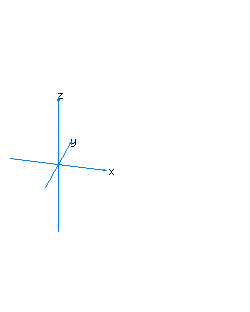1x2+y2+z2+t2=1hypersphere S4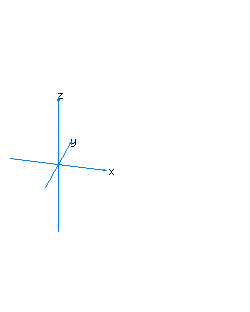2x2+y2+z2-t2=1spherical cone with principal axis on the axis of t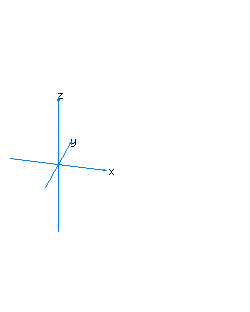3x2+y2-z2+t2=0spherical cone with principal axis on the axis of z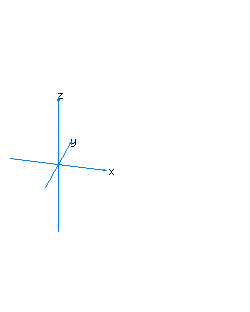4x2-y2+z2+t2=0spherical cone with principal axis on the axis of y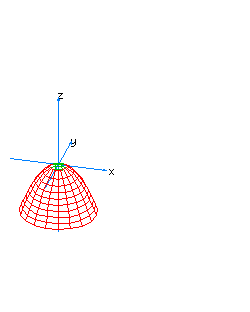5x2+y2-z*t=0spherical cone whose principal axis is the line x=y=z+t=0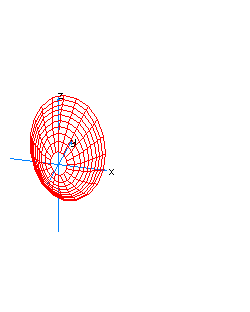6x2+z2-y*t=0spherical cone whose principal axis is the line x=z=y+t=0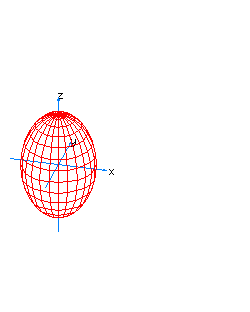7x2+y2+z2-t2=1spherical hyperboloid whose principal axis is the axis of t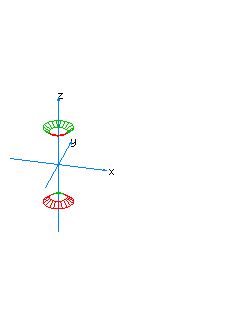8x2+y2-z2+t2=1spherical hyperboloid whose principal axis is the axis of z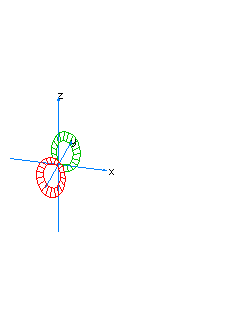9x2-y2+z2+t2=1spherical hyperboloid whose principal axis is the axis of y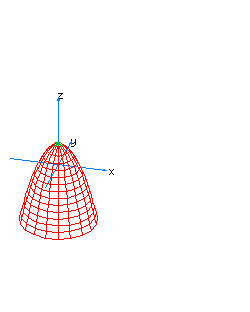10x2+y2-z*t=1spherical hyperboloid whose principal axis is the line x=y=z+t=0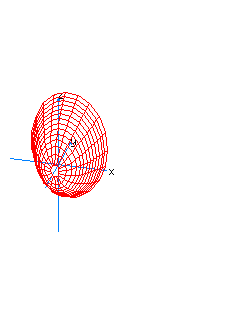11x2+z2-y*t=1spherical hyperboloid whose principal axis is the line x=z=y+t=0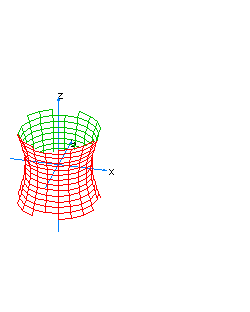12x2+y2-z2-t2=0vertical hyperboloidal cone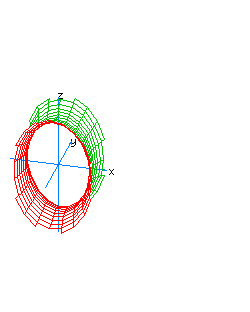13x2-y2+z2-t2=0horizontal hyperboloidal cone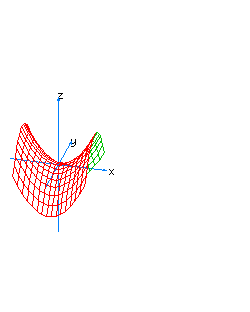14x2-y2-z*t=0hyperboloidal cone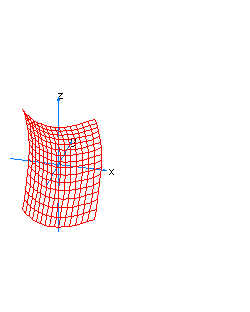15x2-z2-y*t=0hyperboloidal cone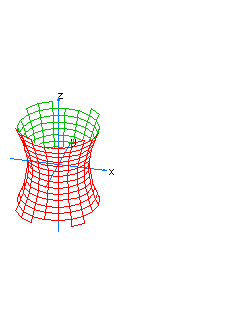16x2+y2-z2-t2=1hyperboloidal hyperboloid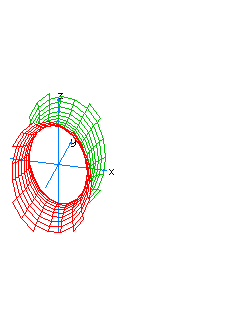17x2-y2+z2-t2=1hyperboloidal hyperboloid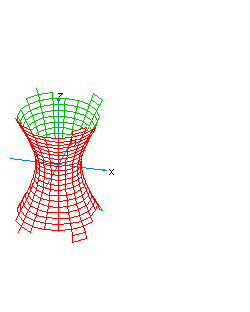18x2+y2-z2-t2= -1hyperboloidal hyperboloid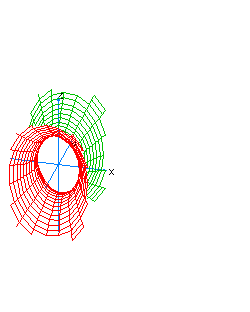19x2-y2+z2-t2= -1hyperboloidal hyperboloid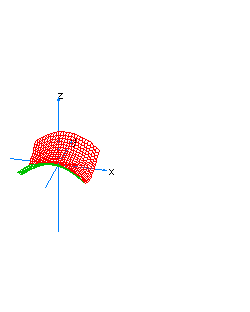20x2-y2-z*t=1hyperboloidal hyperboloid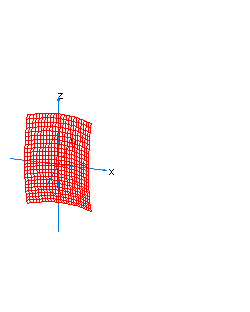21x2-z2-y*t=1hyperboloidal hyperboloid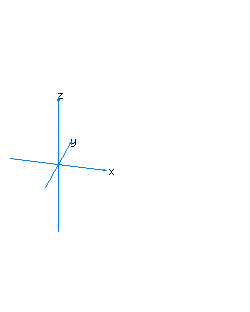22x2+y2+z2-t=0spherical paraboloid oriented towards the axis of t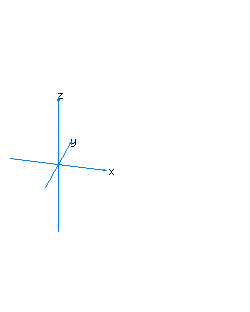23x2+y2-z+t2=0spherical paraboloid oriented towards the axis of z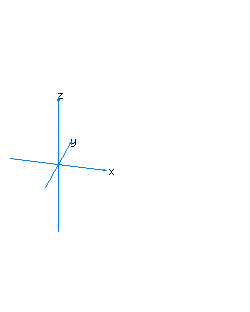24x2-y+z2+t2=0spherical paraboloid oriented towards the axis of y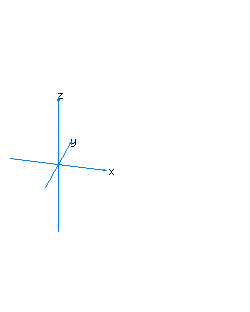25x2+y2-z2-t=0hyperboloidal paraboloid oriented towards the axis of t, vertical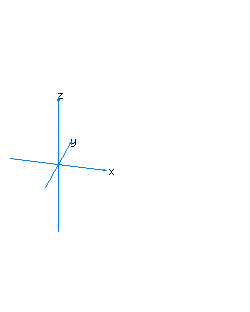26x2-y2+z2-t=0hyperboloidal paraboloid oriented towards the axis of t, horizontal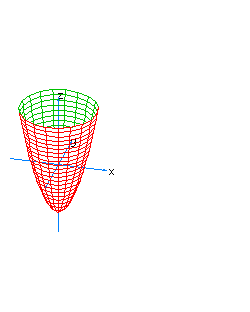27x2+y2-z-t2=0hyperboloidal paraboloid oriented towards the axis of z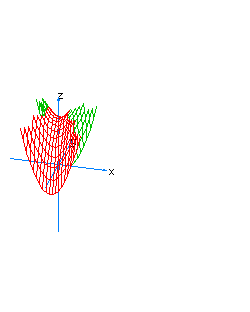28x2-y2-z+t2=0hyperboloidal paraboloid oriented towards the axis of z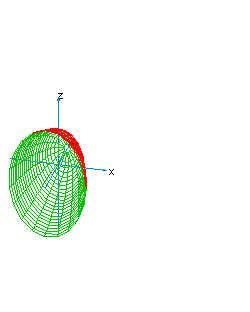29x2-y+z2-t2=0hyperboloidal paraboloid oriented towards the axis of y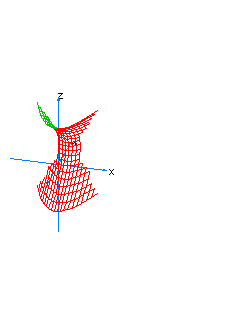30x2-y-z2+t2=0hyperboloidal paraboloid oriented towards the axis of y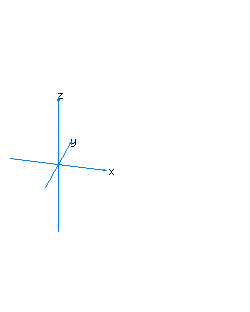31x2+y2+t2=1vertical spherical cylinder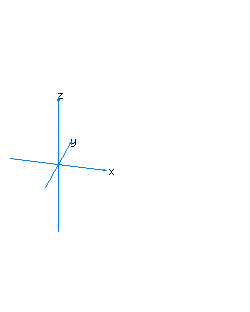32x2+z2+t2=1horizontal spherical cylinder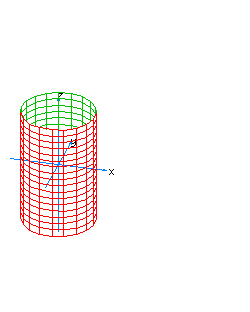33x2+y2-t2=0conic cylinder with a singular line on the axis of z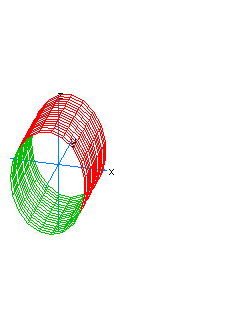34x2+z2-t2=0conic cylinder with a singular line on the axis of y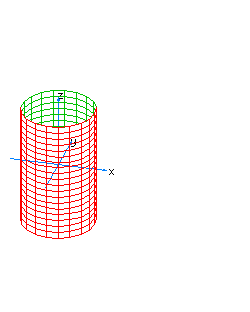35x2+y2-t2=1hyperboloidal cylinder with one sheet, vertical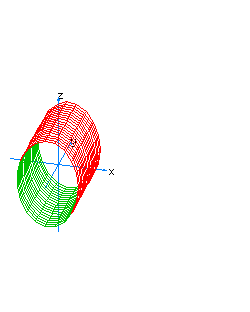36x2+z2-t2=1hyperboloidal cylinder with one sheet, horizontal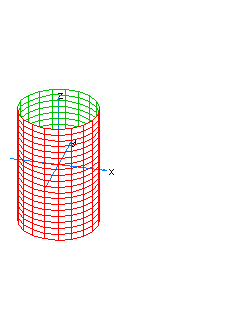37x2+y2-t2= 1hyperboloidal cylinder with two sheets, vertical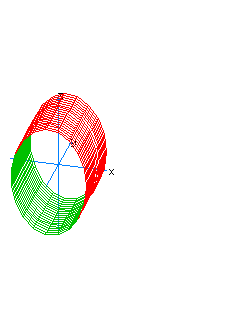38y2+z2-t2= 1hyperboloidal cylinder with two sheets, horizontal
? 39x*y*z-t=0 Dr hypersurface
? 40 z1.im*cos(a) + z2.im*sin(a) Fermat hypersurface

## Reference 参阅

• example 例题
• function graph 制图
• graphics 制图法
• animation 动画 ﻿